# Calculus 3 : Cylindrical Coordinates

## Example Questions

### Example Question #241 : Cylindrical Coordinates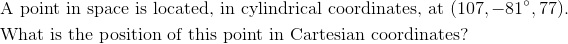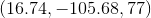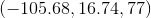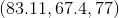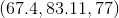Explanation: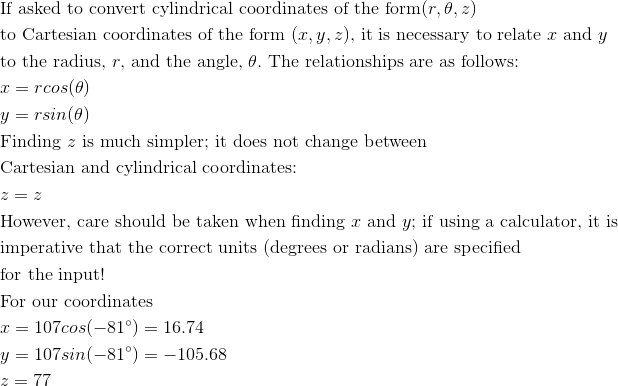### Example Question #241 : Cylindrical Coordinates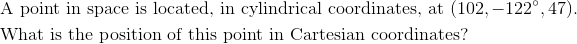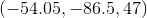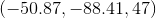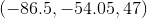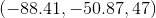Explanation: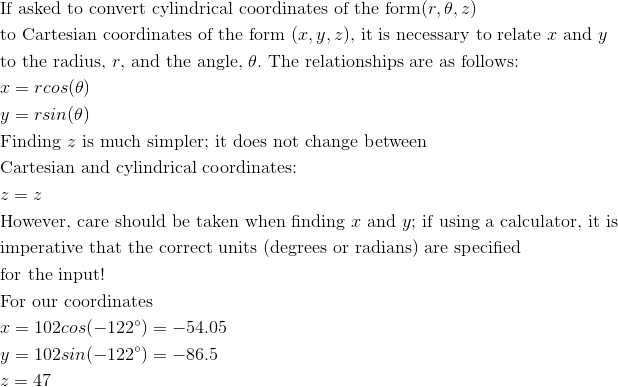### Example Question #241 : Cylindrical Coordinates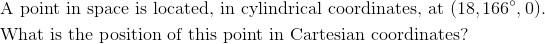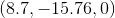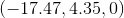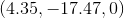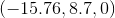Explanation: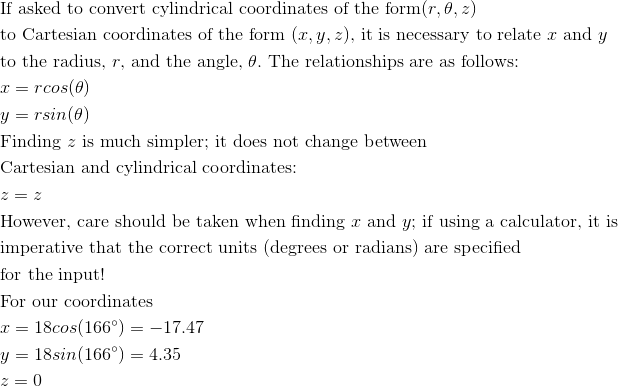### Example Question #244 : Cylindrical Coordinates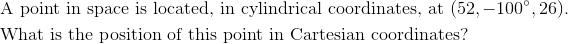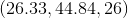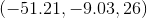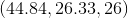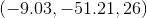Explanation: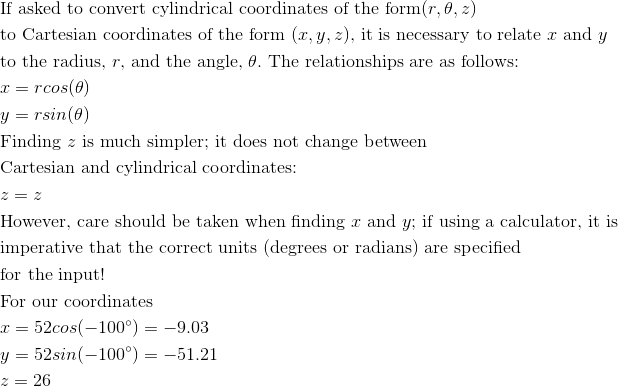### Example Question #245 : Cylindrical Coordinates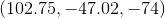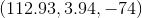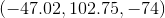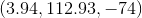Explanation: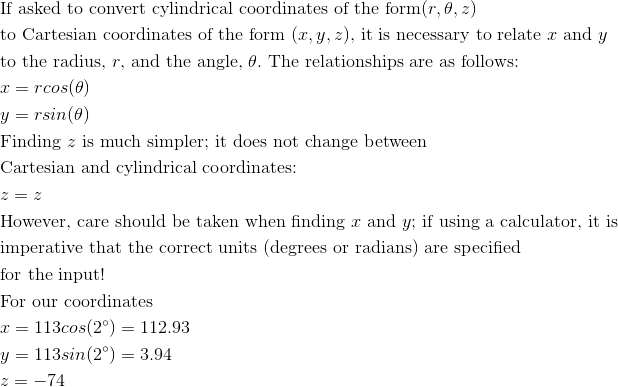### Example Question #331 : 3 Dimensional Space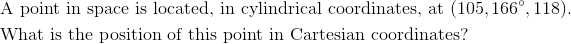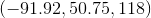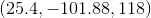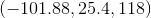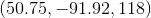Explanation: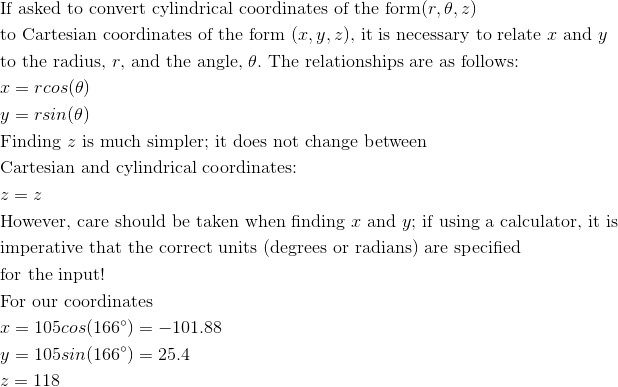### Example Question #332 : 3 Dimensional Space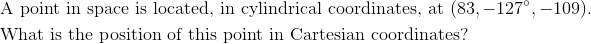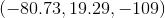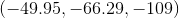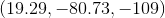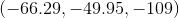Explanation: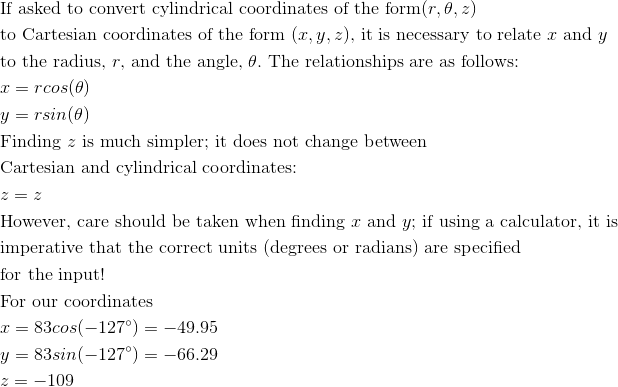### Example Question #248 : Cylindrical Coordinates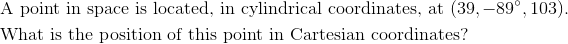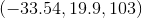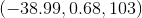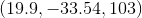Explanation: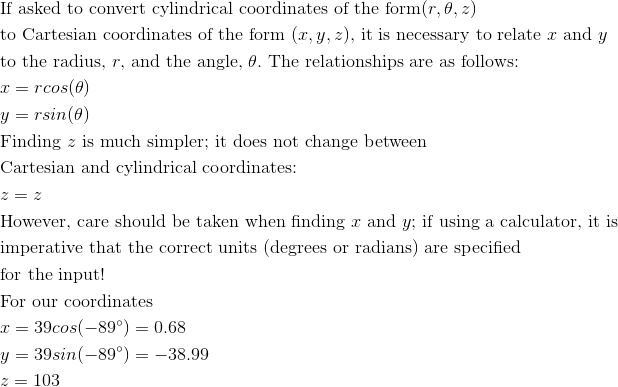### Example Question #249 : Cylindrical Coordinates

Find a parametric representation of the circle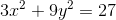.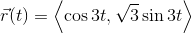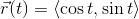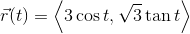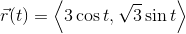Explanation:

We can begin by rewriting the equation for a circle as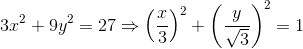.

This directly tells us that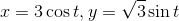.  This allows us to write our final expression for the parametric representation as### Example Question #241 : Cylindrical Coordinates

Convert the following vector in Cartesian coordinates into cylindrical coordinates.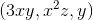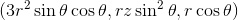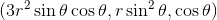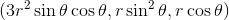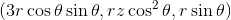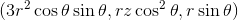Explanation:

The conversion from Cartesian to cylindrical coordinates is as follows: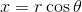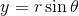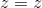The three components of the vector then become: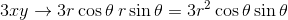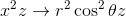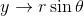### All Calculus 3 Resources